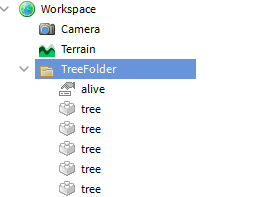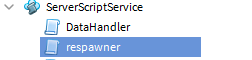# Tree spawning at terrain y level

Hi, i want to make a script that clones trees to a random position. But i don’t know how to make so the tree spawns on the y level matching the terrain level at the position. Any help would be appreciated2 Likes

I want them to to cloned every 60 seconds because you will be able to cut them down.

Just use a small, invisible “test” part before placing the tree.
Move the test part from up to down (x,z is the desired point for the tree) and see when it touches the terrain first, that will be the ground level.

I think that the terrain can have a variation in height

I’d recommend using Rays to achieve this. We can use these rays to find where the ground starts at a given point:

First, we pick some arbitrary search height, like Y=100. If you have terrain higher than this, trees won’t spawn above it. Let’s set up a few constants:

``````math.randomseed(tick()) -- will 'randomize' our pseudo-random number generator
local check_height = 100
local map_x, map_y = 500, 500 -- map size
``````

Now, we use a `for` loop to place as many trees as we want.

``````for i = 1, 500 do -- loops 500 times
local rand_x = math.random()*map_x - map_x/2
local rand_y = math.random()*map_y - map_y/2
end
``````

Next, we create our Ray object with the following syntax:
`Ray Ray.new(Vector3 origin, Vector3 direction)`
Where origin is a Vector3 where the line originates from, and direction is our direction vector (multiplied by a certain amount to have longer rays).

``````local ray = Ray.new(
Vector3.new(rand_x, check_height, rand_y),
Vector3.new(0, -1, 0)*300 -- downwards-facing vector
)
``````

Finally, we can use Workspace:FindPartOnRay [not sure why it’s WorldRoot now] with the following syntax:

``````local inst, position, normal = workspace:FindPartOnRay(ray, ignore_instance, cube_terrain, ignore_water)
``````

Where `inst` is a BasePart or Terrain, position is the Vector3 point of intersection, and normal is the ‘surface direction’ which isn’t needed in this case.

The full code looks as follows:

``````math.randomseed(tick())
local check_height = 100
local map_x, map_y = 500, 500

for i = 1, 500 do
local rand_x = math.random()*map_x - map_x/2
local rand_y = math.random()*map_y - map_y/2

local ray = Ray.new(
Vector3.new(rand_x, check_height, rand_y),
Vector3.new(0, -1, 0)*300
)

local inst, position = workspace:FindPartOnRay(ray, nil, false, true)

if inst and inst:IsA("Terrain") then
place_tree(rand_x, position.y, rand_y) -- not sure what your method is
end
end
``````

If something’s wrong, it’s probably because this was typed on mobile6 Likes

Edit: what a noob I was in 2019

First make sure it’s something like :Here’s what I got, first script in server script service for normal randomization
1] (can result in y errors and cloning out of world,there is a better script I made too below choose which one you want.)

script 1 in serverscript service , :

``````--[[    XxELECTROFUSIONxX
in the script that breaks the tree
add this somewhere  when you destroy the tree

after target(or what you set the tree to):Destroy or breakjoints()
add here : local function destroyed()
that_tree or tree.alive(bool value).Value=false
end

destroyed() so that the value gets set to false, meaning it does not exist currently
then also remember to have one identical tree in lighting too before using this script.
Make sure that there is an intValue called position in everyone of the trees aswell as the bool value mentioned earlier
before you destroy the tree, make sure when it's destroyed, the tree is cloned to another folder called NewTreeFolder  that contains script a, mentioned below and a vector3value that can be set to any value
The NewTreeFolder should be located in Replicated Storage!
also an example ,what to do instead of destroying it
local c = target/tree:Clone()
c.Parent= game.ReplicatedStorage.NewTreeFolder/in that folder there should be a  script, that script is located in my post below this script code.
--]]

local wait_time = 60--time,s,amt
local folder = workspace.TreeFolder
local alive = folder.alive--it's supposed to be a bool value and it should be in every tree , value set to false (un ticked)
local To_Be_Cloned = workspace.TreeFolder:GetChildren()

for _,onetree in pairs (To_Be_Cloned)  do
local onetreeX = onetree
if not onetreeX
then return end
onetreeX.alive.Value=true print("it's alive!..")
onetreeX.Position = Vector3.new(math.random(), math.random(), math.random())--Use this for randomizing position or for an even better method preventing it from going down too much/Y problem,Look at the second script below

end
``````

Script 2 / in replicated storage/in the folder (can be used separately too)

``````local delaytime = 30--after what time it respawns(is cloned to workspace)

local x = script.Parent.Vector3Value
local pos1 = Vector3.new(1,31,13)
local pos2 = Vector3.new(912,324,123)
local pos3 = Vector3.new(93,13,0)
function randompos()

local NewGuy = script.Parent:FindFirstChild("tree")
local m = math.random(1,2)--2 being amt. of possible Positions
if m==1  then x.Value = pos1
if m==2  then x.Value = pos2
if m==3 then x.Value = pos3
end
end
end

wait(delaytime)

end
``````

That’s it, though what @ee0w suggested is a good idea too

1 Like

Nvm, i fixed it. Thank you for the helpIt works
Assuming the terrain surface level is between -10 and 200 (you can change it simply):
x and z can be the desired place of your tree (I use random spots in the middle of the workspace)
“tester” is a 1x1x1 sized block part.
Transparency, CanCollide, Anchored can be any, it doesn’t matter too much, it works in any way.

I put this script inside the tester part, but it works anywhere (change tester object accordingly):

while wait(2) do

``````local x = math.random(-100,100)
local z = math.random(-100,100)
local y
local tester=script.parent

for y=200,-10, -1 do
tester.Position=Vector3.new(x,y,z)
for _, o in pairs(tester:GetTouchingParts()) do
print (y,o.Name, tester.Position)
end
end

tester.Touched:Connect(function() end)
``````

end

1 Like